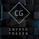11788 vizualizações
Here is an example of a script showing a multi-time frame of TSI.

Chart below compares FX EURUSD Daily TSI to 1H TSI

Here is an updated version

study("True Strength Indicator MTF", shorttitle="TSI MTF")
resCustom = input(title="Timeframe", type=resolution, defval="60" )
long = input(title="Long Length", type=integer, defval=25)
short = input(title="Short Length", type=integer, defval=13)
signal = input(title="Signal Length", type=integer, defval=13)
price = close
double_smooth( src , long, short) =>
fist_smooth = ema ( src , long)
ema (fist_smooth, short)
pc = change(price)
double_smoothed_pc = double_smooth( pc , long, short)
double_smoothed_abs_pc = double_smooth(abs( pc ), long, short)
tsi_value = 100 * (double_smoothed_pc / double_smoothed_abs_pc)
tsi = security(tickerid, resCustom,tsi_value)
plot(tsi, color=black)
plot( ema (tsi, signal), color=red)
hline(0, title="Zero")
```study("True Strength Indicator MTF", shorttitle="TSI MTF")
resCustom = input(title="Timeframe", type=resolution, defval="60" )
long = input(title="Long Length", type=integer, defval=25)
short = input(title="Short Length", type=integer, defval=13)
signal = input(title="Signal Length", type=integer, defval=13)
price = close
double_smooth(src, long, short) =>
fist_smooth = ema(src, long)
ema(fist_smooth, short)
pc = change(price)
double_smoothed_pc = double_smooth(pc, long, short)
double_smoothed_abs_pc = double_smooth(abs(pc), long, short)
tsi_value = 100 * (double_smoothed_pc / double_smoothed_abs_pc)
plot(tsi_value, color=black)
plot(ema(tsi_value, signal), color=red)
hline(0, title="Zero")```

## Comentários6 Buy/Sell Techniques using the TSI Indicator

I have identified no fewer than 6 techniques for using the True Strength Index (TSI) indicator for the accurate generation of Buy/Sell trading decisions.
*
I will present these 6 techniques accompanied by charts and demonstrate their interpretation.
*
The 6 techniques are:
*
1. ZERO Crossover
2. Trend Line Break
3. Positive/Negative Divergence
4. Support/Resistance Line Break
5. Nosebleed/Opposite
6. Moving Average Crossover
*
Let's first begin with the ZERO Crossover, as it demonstrates the wonderfully characteristic 'truth' of the True Strength Index indicator:
*
a.) when the indicator is rising above the ZERO level, price is always also rising.
b.) when the indicator is falling below the ZERO level, price is always also falling.

6 Buy/Sell Techniques using the TSI Indicator
Respostastudy("True Strength Indicator MTF", shorttitle="TSI MTF")
resCustom = input(title="Timeframe", type=resolution, defval="60" )
long = input(title="Long Length", type=integer, defval=25)
short = input(title="Short Length", type=integer, defval=13)
signal = input(title="Signal Length", type=integer, defval=13)
price = close
double_smooth(src, long, short) =>
fist_smooth = ema(src, long)
ema(fist_smooth, short)
pc = change(price)
double_smoothed_pc = double_smooth(pc, long, short)
double_smoothed_abs_pc = double_smooth(abs(pc), long, short)
tsi_value = 100 * (double_smoothed_pc / double_smoothed_abs_pc)
tsi = security(tickerid, resCustom,tsi_value)
plot(tsi, color=black)
plot(ema(tsi, signal), color=red)
hline(0, title="Zero")
RespostaAnother example with lower chart time frame vs higher TSI frames.
Respostahello @QuantitativeExhaustion , I'm trying to work using two time frames but it's not working. Can you please help?
Resposta